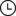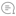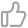【建议收藏】50 道硬核的 Python 面试题！杰哥的IT之旅 |452021-11-26 18:14000

题目001: 在Python中如何实现单例模式。

from functools import wraps

def singleton(cls):
"""单例类装饰器"""
instances = {}

@wraps(cls)
def wrapper(*args, **kwargs):
if cls not in instances:
instances[cls] = cls(*args, **kwargs)
return instances[cls]

return wrapper

@singleton
class President:
pass

class SingletonMeta(type):
"""自定义单例元类"""

def __init__(cls, *args, **kwargs):
cls.__instance = None
super().__init__(*args, **kwargs)

def __call__(cls, *args, **kwargs):
if cls.__instance is None:
cls.__instance = super().__call__(*args, **kwargs)
return cls.__instance

class President(metaclass=SingletonMeta):
pass

a = a ^ b
b = a ^ b
a = a ^ b

a, b = b, a

题目003：写一个删除列表中重复元素的函数，要求去重后元素相对位置保持不变。

def dedup(items):
no_dup_items = []
seen = set()
for item in items:
if item not in seen:
no_dup_items.append(item)
return no_dup_items

def dedup(items):
seen = set()
for item in items:
if item not in seen:
yield item

题目004：假设你使用的是官方的CPython，说出下面代码的运行结果。

a, b, c, d = 1110001000
print(a is b, c is d)

def foo():
e = 1000
f = 1000
print(e is f, e is d)
g = 1
print(g is a)

foo()

True False
True False
True

CPython底层为了进一步提升性能还做了另一个设定，对于同一个代码块中值不在small_ints缓存范围内的整数，如果同一个代码块中已经存在一个值与其相同的整数对象，那么就直接引用该对象，否则创建新的int对象。需要大家注意的是，这条规则对数值型适用，但对字符串则需要考虑字符串的长度，这一点大家可以自行证明。

题目005：Lambda函数是什么，举例说明的它的应用场景。

Lambda函数也叫匿名函数，它是功能简单用一行代码就能实现的小型函数。Python中的Lambda函数只能写一个表达式，这个表达式的执行结果就是函数的返回值，不用写return关键字。Lambda函数因为没有名字，所以也不会跟其他函数发生命名冲突的问题。

fac = lambda x: __import__('functools').reduce(int.__mul__, range(1, x + 1), 1)
gcd = lambda x, y: y % x and gcd(y % x, x) or x

Lambda函数其实最为主要的用途是把一个函数传入另一个高阶函数（如Python内置的filtermap等）中来为函数做解耦合，增强函数的灵活性和通用性。下面的例子通过使用filtermap函数，实现了从列表中筛选出奇数并求平方构成新列表的操作，因为用到了高阶函数，过滤和映射数据的规则都是函数的调用者通过另外一个函数传入的，因此这filtermap函数没有跟特定的过滤和映射数据的规则耦合在一起。

items = 
items = list(map(lambda x: x ** 2, filter(lambda x: x % 2, items)))
print(items)    # [25, 49, 361]

items = 
items = [x ** 2 for x in items if x % 2]
print(items)    # [25, 49, 361]

题目006：说说Python中的浅拷贝和深拷贝。

import pickle

import copy

class PrototypeMeta(type):
"""实现原型模式的元类"""

def __init__(cls, *args, **kwargs):
super().__init__(*args, **kwargs)
# 为对象绑定clone方法来实现对象拷贝
cls.clone = lambda self, is_deep=True: \
copy.deepcopy(self) if is_deep else copy.copy(self)

class Person(metaclass=PrototypeMeta):
pass

p1 = Person()
p2 = p1.clone()                 # 深拷贝
p3 = p1.clone(is_deep=False)    # 浅拷贝

题目007：Python是如何实现内存管理的？

Python提供了自动化的内存管理，也就是说内存空间的分配与释放都是由Python解释器在运行时自动进行的，自动管理内存功能极大的减轻程序员的工作负担，也能够帮助程序员在一定程度上解决内存泄露的问题。以CPython解释器为例，它的内存管理有三个关键点：引用计数、标记清理、分代收集。

typedef struct _object {
Py_ssize_t ob_refcnt;
struct _typeobject *ob_type;
} PyObject;

• 对象被创建

• 对象被引用

• 对象作为参数传入到一个函数中

• 对象作为元素存储到一个容器中

• del语句显示删除对象引用

• 对象引用被重新赋值其他对象

• 一个对象离开它所在的作用域

• 持有该对象的容器自身被销毁

• 持有该对象的容器删除该对象

题目008：说一下你对Python中迭代器和生成器的理解。

class Fib(object):

def __init__(self, num):
self.num = num
self.a, self.b = 01
self.idx = 0

def __iter__(self):
return self

def __next__(self):
if self.idx < self.num:
self.a, self.b = self.b, self.a + self.b
self.idx += 1
return self.a
raise StopIteration()

def fib(num):
a, b = 01
for _ in range(num):
a, b = b, a + b
yield a

题目009：正则表达式的match方法和search方法有什么区别？

match方法是从字符串的起始位置进行正则表达式匹配，返回Match对象或None。search方法会扫描整个字符串来找寻匹配的模式，同样也是返回Match对象或None。

题目010：下面这段代码的执行结果是什么。

def multiply():
return [lambda x: i * x for i in range(4)]

print([m(100for m in multiply()])



def multiply():
return (lambda x: i * x for i in range(4))

print([m(100for m in multiply()])

def multiply():
for i in range(4):
yield lambda x: x * i

print([m(100for m in multiply()])

from functools import partial
from operator import __mul__

def multiply():
return [partial(__mul__, i) for i in range(4)]

print([m(100for m in multiply()])

题目012：用Python代码实现Python内置函数max。

def my_max(*args, key=None, default=None):
"""
获取可迭代对象中最大的元素或两个及以上实参中最大的元素
:param args: 一个可迭代对象或多个元素
:param key: 提取用于元素比较的特征值的函数，默认为None
:param default: 如果可迭代对象为空则返回该默认值，如果没有给默认值则引发ValueError异常
:return: 返回可迭代对象或多个元素中的最大元素
"""

if len(args) == 1 and len(args) == 0:
if default:
return default
else:
raise ValueError('max() arg is an empty sequence')
items = args[0if len(args) == 1 else args
max_elem, max_value = items, items
if key:
max_value = key(max_value)
for item in items:
value = item
if key:
value = key(item)
if value > max_value:
max_elem, max_value = item, value
return max_elem

题目013：写一个函数统计传入的列表中每个数字出现的次数并返回对应的字典。

def count_letters(items):
result = {}
for item in items:
if isinstance(item, (int, float)):
result[item] = result.get(item, 0) + 1
return result

from collections import Counter

def count_letters(items):
counter = Counter(items)
return {key: value for key, value in counter.items() \
if isinstance(key, (int, float))}

题目014：使用Python代码实现遍历一个文件夹的操作。

Python标准库os模块的walk函数提供了遍历一个文件夹的功能，它返回一个生成器。

import os

for path, dir_list, file_list in g:
for dir_name in dir_list:
print(os.path.join(path, dir_name))
for file_name in file_list:
print(os.path.join(path, file_name))

题目015：现有2元、3元、5元共三种面额的货币，如果需要找零99元，一共有多少种找零的方式？

from functools import lru_cache

@lru_cache()
def change_money(total):
if total == 0:
return 1
if total < 0:
return 0
return change_money(total - 2) + change_money(total - 3) + \
change_money(total - 5)

题目016：写一个函数，给定矩阵的阶数n，输出一个螺旋式数字矩阵。

1 2
4 3

1 2 3
8 9 4
7 6 5

def show_spiral_matrix(n):
matrix = [ * n for _ in range(n)]
row, col = 00
num, direction = 10
while num <= n ** 2:
if matrix[row][col] == 0:
matrix[row][col] = num
num += 1
if direction == 0:
if col < n - 1 and matrix[row][col + 1] == 0:
col += 1
else:
direction += 1
elif direction == 1:
if row < n - 1 and matrix[row + 1][col] == 0:
row += 1
else:
direction += 1
elif direction == 2:
if col > 0 and matrix[row][col - 1] == 0:
col -= 1
else:
direction += 1
else:
if row > 0 and matrix[row - 1][col] == 0:
row -= 1
else:
direction += 1
direction %= 4
for x in matrix:
for y in x:
print(y, end='\t')
print()

题目017：阅读下面的代码，写出程序的运行结果。

items = [1234
print([i for i in items if i > 2])
print([i for i in items if i % 2])
print([(x, y) for x, y in zip('abcd', (12345))])
print({x: f'item{x ** 2}' for x in (246)})
print(len({x for x in 'hello world' if x not in 'abcdefg'}))

[3, 4]
[1, 3]
[('a', 1), ('b', 2), ('c', 3), ('d', 4)]
{2: 'item4', 4: 'item16', 6: 'item36'}
6

题目018：说出下面代码的运行结果。

class Parent:
x = 1

class Child1(Parent):
pass

class Child2(Parent):
pass

print(Parent.x, Child1.x, Child2.x)
Child1.x = 2
print(Parent.x, Child1.x, Child2.x)
Parent.x = 3
print(Parent.x, Child1.x, Child2.x)

1 1 1
1 2 1
3 2 3

题目19：说说你用过Python标准库中的哪些模块。

sys跟Python解释器相关的变量和函数，例如：sys.versionsys.exit()
os和操作系统相关的功能，例如：os.listdir()os.remove()
re和正则表达式相关的功能，例如：re.compile()re.search()
math和数学运算相关的功能，例如：math.pimath.emath.cos
logging和日志系统相关的类和函数，例如：logging.Loggerlogging.Handler
hashlib封装了多种哈希摘要算法的模块，例如：hashlib.md5hashlib.sha1
urllib包含了和URL相关的子模块，例如：urllib.requesturllib.parse
itertools提供各种迭代器的模块，例如：itertools.cycleitertools.product
functools函数相关工具模块，例如：functools.partialfunctools.lru_cache
collections / heapq封装了常用数据结构和算法的模块，例如：collections.deque
base64提供BASE-64编码相关函数的模块，例如：bas64.encode
profile / cProfile / pstats和代码性能剖析相关的模块，例如：cProfile.runpstats.Stats
unittest和单元测试相关的模块，例如：unittest.TestCase

题目20：init__和__new方法有什么区别？

Python中调用构造器创建对象属于两阶段构造过程，首先执行__new__方法获得保存对象所需的内存空间，再通过__init__执行对内存空间数据的填充（对象属性的初始化）。__new__方法的返回值是创建好的Python对象（的引用），而__init__方法的第一个参数就是这个对象（的引用），所以在__init__中可以完成对对象的初始化操作。__new__是类方法，它的第一个参数是类，__init__是对象方法，它的第一个参数是对象。

题目21：输入年月日，判断这个日期是这一年的第几天。

def is_leap_year(year):
"""判断指定的年份是不是闰年，平年返回False，闰年返回True"""
return year % 4 == 0 and year % 100 != 0 or year % 400 == 0

def which_day(year, month, date):
"""计算传入的日期是这一年的第几天"""
# 用嵌套的列表保存平年和闰年每个月的天数
days_of_month = [
,

]
days = days_of_month[is_leap_year(year)][:month - 1]
return sum(days) + date

import datetime

def which_day(year, month, date):
end = datetime.date(year, month, date)
start = datetime.date(year, 11)
return (end - start).days + 1

题目22：平常工作中用什么工具进行静态代码分析。

1. 代码是否符合编码规范，例如：PEP-8。

2. 代码中潜在的问题，包括：语法错误、缩进问题、导入缺失、变量覆盖等。

3. 代码中的坏味道。

4. 代码的复杂度。

5. 代码的逻辑问题。

题目23：说一下你知道的Python中的魔术方法。

__new____init____del__创建和销毁对象相关
__eq____ne____lt____gt____le____ge__关系运算符相关
__pos____neg____invert__一元运算符相关
__lshift____rshift____and____or____xor__位运算相关
__enter____exit__上下文管理器协议
__iter____next____reversed__迭代器协议
__int____long____float____oct____hex__类型/进制转换相关
__str____repr____hash____dir__对象表述相关
__len____getitem____setitem____contains____missing__序列相关
__copy____deepcopy__对象拷贝相关
__call____setattr____getattr____delattr__其他魔术方法

题目24：函数参数arg和*kwargs分别代表什么？

Python中，函数的参数分为位置参数、可变参数、关键字参数、命名关键字参数。*args代表可变参数，可以接收0个或任意多个参数，当不确定调用者会传入多少个位置参数时，就可以使用可变参数，它会将传入的参数打包成一个元组。**kwargs代表关键字参数，可以接收用参数名=参数值的方式传入的参数，传入的参数的会打包成一个字典。定义函数时如果同时使用*args**kwargs，那么函数可以接收任意参数。

题目25：写一个记录函数执行时间的装饰器。

from functools import wraps
from time import time

def record_time(func):

@wraps(func)
def wrapper(*args, **kwargs):
start = time()
result = func(*args, **kwargs)
print(f'{func.__name__}执行时间: {time() - start}秒')
return result

return wrapper

from functools import wraps
from time import time

class Record:

def __call__(self, func):

@wraps(func)
def wrapper(*args, **kwargs):
start = time()
result = func(*args, **kwargs)
print(f'{func.__name__}执行时间: {time() - start}秒')
return result

return wrapper

from functools import wraps
from time import time

def record_time(output):
"""可以参数化的装饰器"""

def decorate(func):

@wraps(func)
def wrapper(*args, **kwargs):
start = time()
result = func(*args, **kwargs)
output(func.__name__, time() - start)
return result

return wrapper

return decorate

题目27：说一下Python中变量的作用域。

Python中有四种作用域，分别是局部作用域（Local）、嵌套作用域（Embedded）、全局作用域（Global）、内置作用域（Built-in），搜索一个标识符时，会按照LEGB的顺序进行搜索，如果所有的作用域中都没有找到这个标识符，就会引发NameError异常。

题目30：说一下Python 2和Python 3的区别。

1. Python 2中的printexec都是关键字，在Python 3中变成了函数。

2. Python 3中没有long类型，整数都是int类型。

3. Python 2中的不等号&lt;&gt;在Python 3中被废弃，统一使用!=

4. Python 2中的xrange函数在Python 3中被range函数取代。

5. Python 3对Python 2中不安全的input函数做出了改进，废弃了raw_input函数。

6. Python 2中的file函数被Python 3中的open函数取代。

7. Python 2中的/运算对于int类型是整除，在Python 3中要用//来做整除除法。

8. Python 3中改进了Python 2捕获异常的代码，很明显Python 3的写法更合理。

9. Python 3生成式中循环变量的作用域得到了更好的控制，不会影响到生成式之外的同名变量。

10. Python 3中的round函数可以返回intfloat类型，Python 2中的round函数返回float类型。

11. Python 3的str类型是Unicode字符串，Python 2的str类型是字节串，相当于Python 3中的bytes

12. Python 3中的比较运算符必须比较同类对象。

13. Python 3中定义类的都是新式类，Python 2中定义的类有新式类（显式继承自object的类）和旧式类（经典类）之分，新式类和旧式类在MRO问题上有非常显著的区别，新式类可以使用class__属性获取自身类型，新式类可以使用__slots魔法。

14. Python 3对代码缩进的要求更加严格，如果混用空格和制表键会引发TabError

15. Python 3中字典的keysvaluesitems方法都不再返回list对象，而是返回view object，内置的mapfilter等函数也不再返回list对象，而是返回迭代器对象。

16. Python 3标准库中某些模块的名字跟Python 2是有区别的；而在三方库方面，有些三方库只支持Python 2，有些只能支持Python 3。

题目31：谈谈你对“猴子补丁”（monkey patching）的理解。

“猴子补丁”是动态类型语言的一个特性，代码运行时在不修改源代码的前提下改变代码中的方法、属性、函数等以达到热补丁（hot patch）的效果。很多系统的安全补丁也是通过猴子补丁的方式来实现的，但实际开发中应该避免对猴子补丁的使用，以免造成代码行为不一致的问题。

import json, ujson

json.__name__ = 'ujson'
json.dumps = ujson.dumps

题目32：阅读下面的代码说出运行结果。

class A:
def who(self):
print('A', end='')

class B(A):
def who(self):
super(B, self).who()
print('B', end='')

class C(A):
def who(self):
super(C, self).who()
print('C', end='')

class D(B, C):
def who(self):
super(D, self).who()
print('D', end='')

item = D()
item.who()

1. Python中的MRO（方法解析顺序）。在没有多重继承的情况下，向对象发出一个消息，如果对象没有对应的方法，那么向上（父类）搜索的顺序是非常清晰的。如果向上追溯到object类（所有类的父类）都没有找到对应的方法，那么将会引发AttributeError异常。但是有多重继承尤其是出现菱形继承（钻石继承）的时候，向上追溯到底应该找到那个方法就得确定MRO。Python 3中的类以及Python 2中的新式类使用C3算法来确定MRO，它是一种类似于广度优先搜索的方法；Python 2中的旧式类（经典类）使用深度优先搜索来确定MRO。在搞不清楚MRO的情况下，可以使用类的mro方法或mro属性来获得类的MRO列表。

2. super()函数的使用。在使用super函数时，可以通过super(类型, 对象)来指定对哪个对象以哪个类为起点向上搜索父类方法。所以上面B类代码中的super(B, self).who()表示以B类为起点，向上搜索self（D类对象）的who方法，所以会找到C类中的who方法，因为D类对象的MRO列表是D --&gt; B --&gt; C --&gt; A --&gt; object

ACBD

题目33：编写一个函数实现对逆波兰表达式求值，不能使用Python的内置函数。

import operator

class Stack:
"""栈（FILO）"""

def __init__(self):
self.elems = []

def push(self, elem):
"""入栈"""
self.elems.append(elem)

def pop(self):
"""出栈"""
return self.elems.pop()

@property
def is_empty(self):
"""检查栈是否为空"""
return len(self.elems) == 0

def eval_suffix(expr):
"""逆波兰表达式求值"""
operators = {
'-': operator.sub,
'*': operator.mul,
'/': operator.truediv
}
stack = Stack()
for item in expr.split():
if item.isdigit():
stack.push(float(item))
else:
num2 = stack.pop()
num1 = stack.pop()
stack.push(operators[item](num1, num2))
return stack.pop()

题目34：Python中如何实现字符串替换操作？

Python中实现字符串替换大致有两类方法：字符串的replace方法和正则表达式的sub方法。

message = 'hello, world!'
print(message.replace('o''O').replace('l''L').replace('he''HE'))

import re

message = 'hello, world!'
pattern = re.compile('[aeiou]')
print(pattern.sub('#', message))

题目35：如何剖析Python代码的执行性能？

example.py

import cProfile

def is_prime(num):
for factor in range(2, int(num ** 0.5) + 1):
if num % factor == 0:
return False
return True

class PrimeIter:

def __init__(self, total):
self.counter = 0
self.current = 1
self.total = total

def __iter__(self):
return self

def __next__(self):
if self.counter < self.total:
self.current += 1
while not is_prime(self.current):
self.current += 1
self.counter += 1
return self.current
raise StopIteration()

cProfile.run('list(PrimeIter(10000))')

@profiler
def is_prime(num):
for factor in range(2, int(num ** 0.5) + 1):
if num % factor == 0:
return False
return True

pip install line_profiler

kernprof -lv example.py

Line #    Hits    Time      Per Hit  % Time  Line Contents
==============================================================
1                                       @profile
2                                       def is_prime(num):
3    86624   48420.0   0.6      50.5        for factor in range(2, int(num ** 0.5) + 1):
4    85624   44000.0   0.5      45.9            if num % factor == 0:
5    6918     3080.0   0.4       3.2                return False
6    1000      430.0   0.4       0.4        return True

题目36：如何使用random模块生成随机数、实现随机乱序和随机抽样？

1. random.random()函数可以生成[0.0, 1.0)之间的随机浮点数。

2. random.uniform(a, b)函数可以生成[a, b][b, a]之间的随机浮点数。

3. random.randint(a, b)函数可以生成[a, b][b, a]之间的随机整数。

4. random.shuffle(x)函数可以实现对序列x的原地随机乱序。

5. random.choice(seq)函数可以从非空序列中取出一个随机元素。

6. random.choices(population, weights=None, *, cum_weights=None, k=1)函数可以从总体中随机抽取（有放回抽样）出容量为k的样本并返回样本的列表，可以通过参数指定个体的权重，如果没有指定权重，个体被选中的概率均等。

7. random.sample(population, k)函数可以从总体中随机抽取（无放回抽样）出容量为k的样本并返回样本的列表。

题目37：解释一下线程池的工作原理。

1. 线程池管理器：用于创建并管理线程池。

2. 工作线程和线程队列：线程池中实际执行的线程以及保存这些线程的容器。

3. 任务接口：将线程执行的任务抽象出来，形成任务接口，确保线程池与具体的任务无关。

4. 任务队列：线程池中保存等待被执行的任务的容器。

题目39：说出下面代码的运行结果。

def extend_list(val, items=[]):
items.append(val)
return items

list1 = extend_list(10)
list2 = extend_list(123, [])
list3 = extend_list('a')
print(list1)
print(list2)
print(list3)

[10, 'a']

[10, 'a']

题目40：如何读取大文件，例如内存只有4G，如何读取一个大小为8G的文件？

with open('...''rb'as file:
for data in iter(lambda: file.read(2097152), b''):
pass

split -b 512m filename

split -n 10 filename

题目42：说一下你知道的Python编码规范。

1. 空格的使用

• 使用空格来表示缩进而不要用制表符（Tab）。

• 和语法相关的每一层缩进都用4个空格来表示。

• 每行的字符数不要超过79个字符，如果表达式因太长而占据了多行，除了首行之外的其余各行都应该在正常的缩进宽度上再加上4个空格。

• 函数和类的定义，代码前后都要用两个空行进行分隔。

• 在同一个类中，各个方法之间应该用一个空行进行分隔。

• 二元运算符的左右两侧应该保留一个空格，而且只要一个空格就好。

1. 标识符命名

• 变量、函数和属性应该使用小写字母来拼写，如果有多个单词就使用下划线进行连接。

• 类中受保护的实例属性，应该以一个下划线开头。

• 类中私有的实例属性，应该以两个下划线开头。

• 类和异常的命名，应该每个单词首字母大写。

• 模块级别的常量，应该采用全大写字母，如果有多个单词就用下划线进行连接。

• 类的实例方法，应该把第一个参数命名为self以表示对象自身。

• 类的类方法，应该把第一个参数命名为cls以表示该类自身。

1. 表达式和语句

• 采用内联形式的否定词，而不要把否定词放在整个表达式的前面。例如：if a is not b就比if not a is b更容易让人理解。

• 不要用检查长度的方式来判断字符串、列表等是否为None或者没有元素，应该用if not x这样的写法来检查它。

• 就算if分支、for循环、except异常捕获等中只有一行代码，也不要将代码和ifforexcept等写在一起，分开写才会让代码更清晰。

• import语句总是放在文件开头的地方。

• 引入模块的时候，from math import sqrtimport math更好。

• 如果有多个import语句，应该将其分为三部分，从上到下分别是Python标准模块第三方模块自定义模块，每个部分内部应该按照模块名称的字母表顺序来排列。

题目43：运行下面的代码是否会报错，如果报错请说明哪里有什么样的错，如果不报错请说出代码的执行结果。

class A:
def __init__(self, value):
self.__value = value

@property
def value(self):
return self.__value

obj = A(1)
obj.__value = 2
print(obj.value)
print(obj.__value)

1
2

题目44：对下面给出的字典按值从大到小对键进行排序。

prices = {
'AAPL'191.88,
'GOOG'1186.96,
'IBM'149.24,
'ORCL'48.44,
'ACN'166.89,
'FB'208.09,
'SYMC'21.29
}

sorted(prices, key=lambda x: prices[x], reverse=True

题目45：说一下namedtuple的用法和作用。

from collections import namedtuple

Card = namedtuple('Card', ('suite''face'))
card1 = Card('红桃'13)
card2 = Card('草花'5)
print(f'{card1.suite}{card1.face}')
print(f'{card2.suite}{card2.face}')

class MyCard(Card):

def show(self):
faces = ['''A''2''3''4''5''6''7''8''9''10''J''Q''K']
return f'{self.suite}{faces[self.face]}'

print(Card)    # <class '__main__.Card'>
card3 = MyCard('方块'12)
print(card3.show())    # 方块Q
print(dict(card1._asdict()))    # {'suite': '红桃', 'face': 13}
print(card2._replace(suite='方块'))    # Card(suite='方块', face=5)

题目46：按照题目要求写出对应的函数。

def more_than_half(items):
temp, times = None0
for item in items:
if times == 0:
temp = item
times += 1
else:
if item == temp:
times += 1
else:
times -= 1
return temp

题目47：按照题目要求写出对应的函数。

def list_depth(items):
if isinstance(items, list):
max_depth = 1
for item in items:
max_depth = max(list_depth(item) + 1, max_depth)
return max_depth
return 0

题目48：按照题目要求写出对应的装饰器。

from functools import wraps
from random import random
from time import sleep

def retry(*, retry_times=3, max_wait_secs=5, errors=(Exception, )):

def decorate(func):

@wraps(func)
def wrapper(*args, **kwargs):
for _ in range(retry_times):
try:
return func(*args, **kwargs)
except errors:
sleep(random() * max_wait_secs)
return None

return wrapper

return decorate

from functools import wraps
from random import random
from time import sleep

class Retry(object):

def __init__(self, *, retry_times=3, max_wait_secs=5, errors=(Exception, )):
self.retry_times = retry_times
self.max_wait_secs = max_wait_secs
self.errors = errors

def __call__(self, func):

@wraps(func)
def wrapper(*args, **kwargs):
for _ in range(self.retry_times):
try:
return func(*args, **kwargs)
except self.errors:
sleep(random() * self.max_wait_secs)
return None

return wrapper

题目49：写一个函数实现字符串反转，尽可能写出你知道的所有方法。

def reverse_string(content):
return content[::-1]

def reverse_string(content):
return ''.join(reversed(content))

def reverse_string(content):
if len(content) <= 1:
return content
return reverse_string(content[1:]) + content

from collections import deque

def reverse_string(content):
q = deque()
q.extendleft(content)
return ''.join(q)

from io import StringIO

def reverse_string(content):
buffer = StringIO()
for i in range(len(content) - 1-1-1):
buffer.write(content[i])
return buffer.getvalue()

def reverse_string(content):
return ''.join([content[i] for i in range(len(content) - 1-1-1)])

def reverse_string(content):
length, content= len(content), list(content)
for i in range(length // 2):
content[i], content[length - 1 - i] = content[length - 1 - i], content[i]
return ''.join(content)

def reverse_string(content):
length, content= len(content), list(content)
for i, j in zip(range(length // 2), range(length - 1, length // 2 - 1-1)):
content[i], content[j] = content[j], content[i]
return ''.join(content)

题目50：按照题目要求写出对应的函数。

def find_dup(items: list):
dups =  * 9000
for item in items:
dups[item - 1000] += 1
for idx, val in enumerate(dups):
if val > 1:
yield idx + 1000

https://github.com/jackfrued/Python-Interview-Bible/blob/master/Python%E9%9D%A2%E8%AF%95%E5%AE%9D%E5%85%B8-%E5%9F%BA%E7%A1%80%E7%AF%87-2020.md

推荐阅读

MySQL 高频面试题！

78 道大厂 MongoDB 面试题集锦！

23 道 Kubernetes 面试题！00

暂无评论~~
Ctrl+Enter